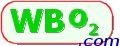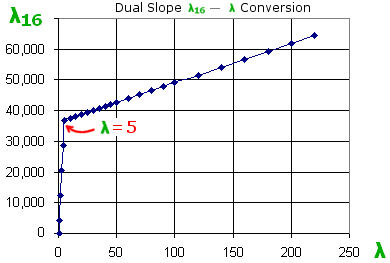# Lambda-16 (λ16) - High Resolution AFR/Lambda ValueThis page describes λ16. For WBo2 wideband firmware xx61 (and higher) the RS232 2.0 frame format is modified. The Wideband ADC value (bytes 6 and 7) is changed to be Lambda-16 (abbreviated in normal text to L16 or to λ16 in formatted text).### λ16 ⇔ λ - Formula and Conversions

Lambda-16 is an unsigned 16 bit number representing lambda (λ). For simplicity and to preserve accuracy across the possible range of Lambda, Lambda-16 is a dual-slope transfer function covering the ranges :

1. Lambda = 0.5 → 5.0 (normal usage range of unleaded AFR = 7.35 to AFR = 73.5)
2. Lambda = 5.0 → free-air (useful for non-tuning wideband applications)

Lambda (λ) is converted to Lambda-16 (λ16) as follows

```   If (λ < 5.0)
λ16 = ( λ - 0.5 ) * 8192
else
λ16 = 36864 + (( λ - 5.0 ) * 128)
```

Lambda-16 is converted to Lambda as follows:

```    if (λ16 < 36864)
λ = ( λ16 / 8192 ) + 0.5
else
λ = 5.0 + ((λ16 - 36864 ) / 128)
```

Note that AFR is directly related to λ, and λ16, by

AFR = λ * AFRstoich
AFR = (( λ16 / 8192 ) + 0.5 ) * AFRstoich         (for the useful vehicle tuning range)

Where AFRstoich is the AFR value at stoich for the fuel used (14.7 for unleaded).

### λ16 Precision

For lambda < 5.0 the granularity of Lambda derived from Lambda-16 is (1/8192) = 0.000122 so for Lambda between 0.500 and 5.000 it is represented very precisely to 3 decimal places.

For Lambda > 5.0 the granularity is (1/128) = .0078 so a 1 (or closer to 1.5) decimal place representation for Lambda is very precise.

The limits for the Lambda-16's representation of Lambda are 0.5 (L16 = 0) and Lambda = 224.0 (L16 = 65,535).

### % Oxygen, λ and Wideband

Bosch have a (rough) formula for calculating λ from percent oxygen (xO2) - it only works in the lean region where % oxygen is positive, and it also assumes the H/C (Hydrogen to Carbon) ratio is 2.0. Here K is a constant and, depending on the Bosch document, is either 4.76 or 4.77 (it depends on what you define as the free-air oxygen concentration). Also xO2 is a fraction, eg. 0.207 for free-air:

• xO2 = 3 * (λ - 1) / (1 + 3 * K * λ)

• λ = (xO2 / 3 + 1) / (1 - K * xO2)

Note that Ipx (see below) is fairly linear when graphed against xO2. Ipx graphed against λ is very non-linear and they actually have a reciprocal relationship.

### Why λ16?

The original Tech Edge wideband controller (the 2A0) worked with just one sensor family (the LSU 4.0/4.2). We didn't think of all future possibilities when we made some firmware design decisions. For RS232 logging we specified a 2.0 data frame with two Lambda representations:

• Ipx = the sensor's Normalized Pump Current where 0 is the maximum rich value and 8192 is the free-air value (ie. Oxygen % = 20.7 and there are no additional hydrocarbons present). Ipx is limited to the range 0 to a slightly larger value than 8192. Ipx at stoich is a value around zero pump current (or close to 4096). The actual stoich Ipx depends on the sensor and to some extent the gas mix.

• DAC-Count = the 12 bit value of the currently programmed WBlin voltage sent to the controller's hardware DAC (Digital to Analogue Converter). For the default output values, the DAC-Count was 0 for AFR = 9.00 and 4095 for AFR = 19.0.

This created some future problems:

• If you use Ipx then non-linear tables and interpolation are, for many people, apparently difficult to program as it requires a non-linear lookup-up table and interpolation, to generate λ (or AFR).

• if the WBlin voltage was re-programmed then the DAC count changes too!

• Different sensors have slightly different Ipx vs. λ curves, which means that if you use the Ipx field and a fixed lookup table, then if the sensor type changes (eg. from LSU 4.0/4.2 to LSU 4.9 or to NTK) then the lookup table should also change.

We wanted to resolve these problem, which were not significant except for those customers who wanted the best possible accuracy our equipment was capable of (and who doesn't). So, we introduced Lambda-16 and put it in the DAC-Count field which was most likely to change anyway.